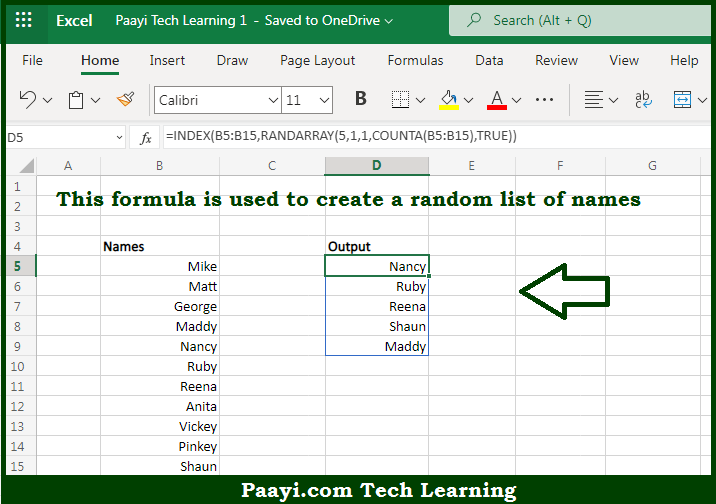# Learn How to Get Random List of Names in Microsoft Excel

Written by | 0 Comments | 533 Views

In this article, you will learn how to evaluate things in Dynamic Arrays with formulas in Microsoft Excel using a single/combination(s) of functions. You will also know How to Get Random List of Names and see the generic formula.

Learn How to Get Random List of Names in Microsoft Excel

The main purpose of this formula is to create a random list of names. Here we will learn how to get the random list of names in the given data range in the workbook in Microsoft Excel. That implies, with the help of formula based on the INDEX, and RANDARRAY functions you can able to create a random list of names. So, with the help of this formula, you can able to get get the random list of names in the given data range in the workbook in Microsoft Excel.

General Formula to Get-Random List of Names

=INDEX(names,RANDARRAY(n,1,1,COUNTA(names),TRUE))

The Explanation to Get Random List of NamesSo we know that with the help of the given formula above you can able to create a random list of names. Here we will learn how to get the random list of names in the given data range in the workbook in Microsoft Excel. As we know that this formula uses the INDEX function to retrieve 10 random names from a named range. The COUNTA function is used to get a dynamic count of names in the given list. So, with the help of this formula, you can able to create a random list of names. So now you have learned how to get the random list of names in the given data range in the workbook in Microsoft Excel.